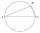# Nonagon

Calculate the area and perimeter of a regular nonagon if its radius of inscribed circle is r = 10cm

Result

S =  327.573 cm2
o =  65.515 cm

#### Solution:Leave us a comment of example and its solution (i.e. if it is still somewhat unclear...):

Showing 0 comments:Be the first to comment!#### To solve this example are needed these knowledge from mathematics:

Pythagorean theorem is the base for the right triangle calculator. See also our trigonometric triangle calculator.

## Next similar examples:

1. PentagonCalculate the length of side, circumference and area of a regular pentagon, which is inscribed in a circle with radius r = 6 cm.
2. DecagonCalculate the area and circumference of the regular decagon when its radius of a circle circumscribing is R = 1m
3. RT 11Calculate the area of right tirangle if its perimeter is p = 45 m and one cathethus is 20 m long.
4. CalculateCalculate the length of a side of the equilateral triangle with an area of 50cm2.
5. TriangleCalculate the area of right triangle ΔABC, if one leg is long 14 and its opposite angle is 59°.
6. Perimeter of triangleIn triangle ABC angle A is 60° angle B is 90° side size c is 15 cm. Calculate the triangle circumference.
7. Isosceles IVIn an isosceles triangle ABC is |AC| = |BC| = 13 and |AB| = 10. Calculate the radius of the inscribed (r) and described (R) circle.
8. ABS CNCalculate the absolute value of complex number -15-29i.
9. 30-gonAt a regular 30-gon the radius of the inscribed circle is 15cm. Find the "a" side size, circle radius "R", circumference, and content area.
10. Trapezoid - RRFind the area of the right angled trapezoid ABCD with the right angle at the A vertex; a = 3 dm b = 5 dm c = 6 dm d = 4 dm
11. Length ITFind the length (circumference) of an isosceles trapezoid in which the length of the bases a,c and the height h are given: a = 8 cm c = 2 cm h = 4 cm
12. Circle annulusThere are 2 concentric circles in the figure. Chord of larger circle 10 cm long is tangent to the smaller circle. What are does annulus have?
13. If theIf the tangent of an angle of a right angled triangle is 0.8. Then its longest side is. .. .
14. Theorem proveWe want to prove the sentense: If the natural number n is divisible by six, then n is divisible by three. From what assumption we started?
15. MapleMaple peak is visible from a distance 3 m from the trunk from a height of 1.8 m at angle 62°. Determine the height of the maple.
16. Triangle ABCIn a triangle ABC with the side BC of length 2 cm The middle point of AB. Points L and M split AC side into three equal lines. KLM is isosceles triangle with a right angle at the point K. Determine the lengths of the sides AB, AC triangle ABC.
17. In a 2In a thirteen sided polygon, the sum of five angles is 1274°, four of the eight angles remaining are equal and the other four are 18° less than each of the equal angles. Find the angles. .